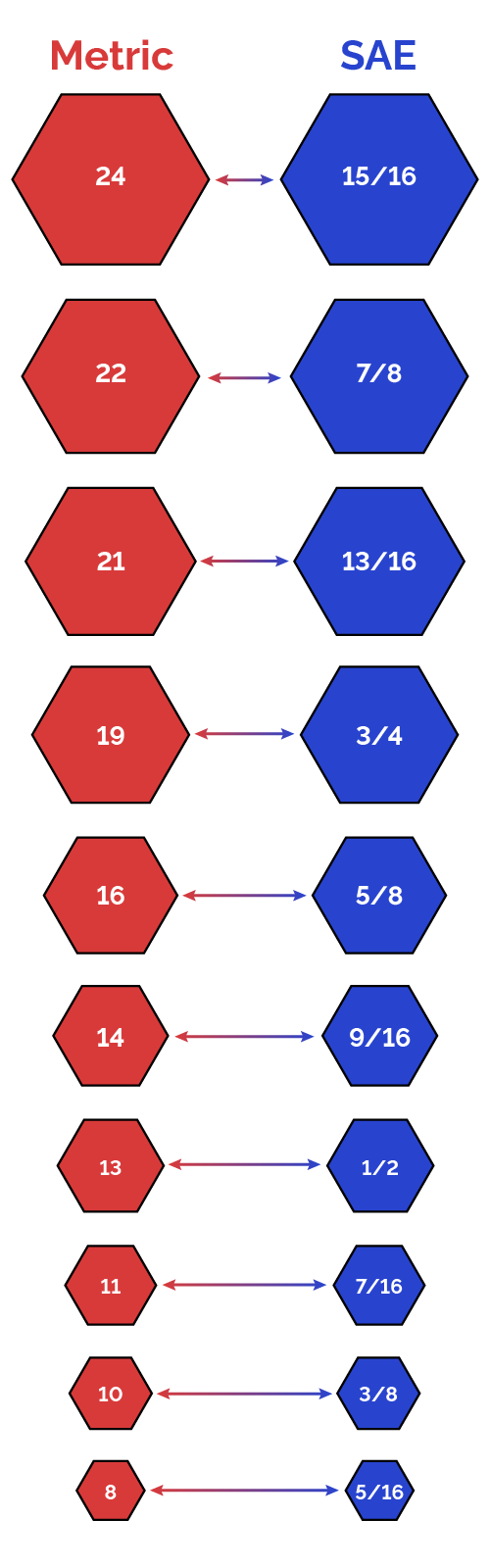# Metric to SAE Calculator

Created by Davide Borchia
Reviewed by Anna Szczepanek, PhD
Last updated: Jan 18, 2023

Our metric to SAE calculator will be a fundamental tool in your arsenal: you will learn how to quickly convert between metric to SAE, a critical knowledge in any workshop and engineering environment. Has it ever happened to you to start tightening a bolt just to find out that your wrench was not fitting perfectly? If yes, you were probably trying to use a metric wrench with an SAE bolt or vice-versa. Keep reading our article to learn:

• What is the SAE system of measurement;
• How to calculate the conversion from metric to SAE units;
• The metric to SAE chart for inches and millimeters.

## What is the SAE system?

The SAE system of measurement units is a collection of measurement units, a subsystem of the imperial system used by American engineers, mechanics, and hobbyists: SAE stands for society of automotive engineers.

SAE units are widely used to measure machines, mechanical components, screws, and bolts. A measurement in SAE units corresponds to a measurement in inches (most often) or feet but is almost always expressed as fraction: in the following sections we will teach you how to calculate the conversion from metric to SAE, and we will show you the metric to SAE chart for a quick, at-a-glance conversion of bolt sizes and much more!

## Calculate the conversion from metric to SAE

To convert from metric to SAE, you only need to learn a simple conversion between metric and imperial units. In most situations, you will have to convert millimeters to inches since these are the units most commonly used:

$1\ \mathrm{mm} = 0.03937\ \mathrm{in}$

You can calculate the metric to SAE conversion between other units by using the following rules:

$1\ \mathrm{in} = \frac{1}{12}\ \mathrm{ft} = \frac{1}{12}\cdot\frac{1}{3}\ \mathrm{yd}$

The story is different (and easier) within the metric system:

$1\ \mathrm{mm} = \frac{1}{10}\ \mathrm{cm} = \frac{1}{1000}\ \mathrm{m}$

However, the result of this conversion is not exactly what we need in most situations! Bolt, nut, and screw sizes come in standardized sizes: $3/4"$, $3/8"$, and $8\ \mathrm{mm}$, and so on; to understand quickly which wrench to use you may have more luck using a metric to SAE chart.

## The metric to SAE chart

Our calculator for the metric to SAE conversion gives you the result you deserve but not the result you need. In your toolbox, you will find wrenches and keys of slightly different sizes, often marked with neat ratios like $3/4$ and $15/16$. Using a metric to SAE chart, you can quickly find the best fitting SAE wrench for a metric bolt, and vice-versa. Here is it:## Other metric and imperial converters

Discover more conversion between and within the imperial and metric systems with our suite of dedicated tools:

## FAQ

### Can I use a metric wrench on a SAE bolt?

Yes! In most cases, you can use a metric wrench on an SAE bolt and vice-versa: the difference in size is often minimal. However, be careful: slightly larger wrenches can deform, wear, and even strip a bolt. It's always better to use the right key!

### How do I convert from metric to SAE?

To calculate the conversion from metric to SAE, follow these easy steps:

1. Measure the size of the bolt in millimeters.
2. Multiply the size in millimeters by 0.03937 to find the size in inches.
3. Find a ratio with a result similar to the conversion you performed. The most used denominators are powers of 2.
4. You can also use a conversion chart between metric and SAE: you will save some time!

### What is 19 mm in SAE?

19 mm equals 0.748 in. However, you will not find such a tool in your toolbox. The correct wrench size is 3/4 in: this tool would be slightly larger than what you need, but it would not matter much!

### What are the most common conversions form metric to SAE?

In the next list you can find the most common conversions between metric and SAE:

• 24 mm = 15/16 in
• 22 mm = 7/8 in
• 21 mm = 13/16 in
• 19 mm = 3/4 in
• 16 mm = 5/8 in
• 14 mm = 9/16 in
• 13 mm = 1/2 in
• 11 mm = 7/16 in
• 10 mm = 3/8 in
• 8 mm = 5/16 in
Davide Borchia
Metric
mm
cm
SAE
in
ft
People also viewed…

### Black Friday

How to get best deals on Black Friday? The struggle is real, let us help you with this Black Friday calculator!

### Car heat

The hot car calculator shows how fast a car's interior heats up during a summer day.

### Degrees minutes seconds

Degrees minutes seconds calculator is a tool that can convert decimal degrees to degrees with minutes and seconds and the other way around.

### Length converter

Length converter enables quick conversion between length units from imperial and metric systems.